Chapter 12, Problem 52GQ

Chapter
Section
Textbook Problem

The solid-state structure of silicon carbide is shown below. (a) How many atoms of each type are contained within the unit cell? What is the formulas of silicon carbide? (b) Knowing that the Si—C bond length is 188.8 pm (and the Si—C—Si bond angle is 109.5°), calculate the density of SiC.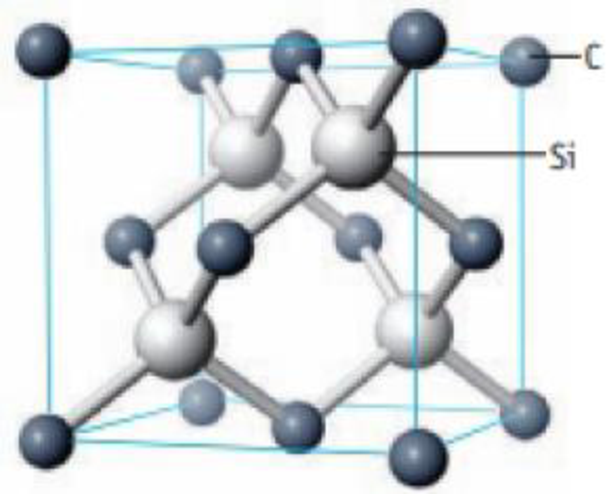Unit cell of SiC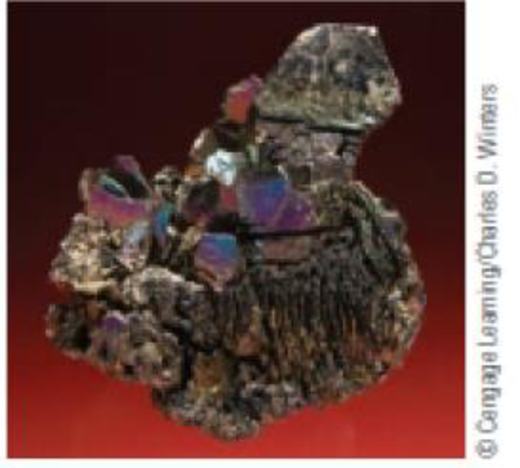Sample of silicon carbide

(a)

Interpretation Introduction

Interpretation:

Number of atoms of each type that are contained within the unit cell and formulas of silicon carbide has to be determined.

Explanation

From the illustration given,

Figure 1

The  atoms at six faces is,

The  atoms at eight corners is,

Total

(b)

Interpretation Introduction

Interpretation:

The density ofhas to be calculated.

Concept introduction:

The volume of the atom is given below,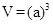The density of the unit cell is calculated as follows,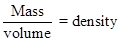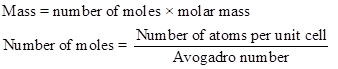Still sussing out bartleby?

Check out a sample textbook solution.

See a sample solution

The Solution to Your Study Problems

Bartleby provides explanations to thousands of textbook problems written by our experts, many with advanced degrees!

Get Started